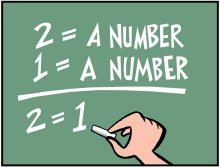# Mathematical Fallacy 4:Is $0=1$?;A DiscussionWe know that $({a}+{b})^2={a}^2+2{a}{b}+{b}^2.$ Using the above identity,we proceed to prove an impossible task:Proving $0=1$!

Try to figure out the fallacy in the below set of equations.Here we go:

\begin{aligned} (n+1)^2=&n^2+2n+1 \quad & \ldots (1) \\ (n+1)^2-(2n+1)=&n^2 \quad & \ldots (2) \\ (n+1)^2-(2n+1)-n(2n+1)=&n^2-n(2n+1) \quad & \ldots (3) \\ (n+1)^2-(2n+1)(n+1)=& n^2-n(2n+1) \quad & \ldots(4) \\ (n+1)^2-(2n+1)(n+1)+\left(\dfrac{2n+1}{2}\right)^2=& n^2-n(2n+1)+\left(\dfrac{2n+1}{2}\right)^2 \quad & \ldots(5) \\ \left[(n+1)-\dfrac{2n+1}{2}\right]^2=&\left[n-\dfrac{2n+1}{2}\right]^2 \quad & \ldots(6) \\ (n+1)-\dfrac{2n+1}{2}=& n-\dfrac{2n+1}{2} \quad & \ldots(7) \\ n+1=& n \quad & \ldots (8) \\ n-n=& 1 \quad & \ldots(9) \\ 0=&1 \quad & \ldots(10) \end{aligned}

Could you figure it out? What do you think? Comment below!Note by Rohit Udaiwal
5 years, 5 months ago

This discussion board is a place to discuss our Daily Challenges and the math and science related to those challenges. Explanations are more than just a solution — they should explain the steps and thinking strategies that you used to obtain the solution. Comments should further the discussion of math and science.

When posting on Brilliant:

• Use the emojis to react to an explanation, whether you're congratulating a job well done , or just really confused .
• Ask specific questions about the challenge or the steps in somebody's explanation. Well-posed questions can add a lot to the discussion, but posting "I don't understand!" doesn't help anyone.
• Try to contribute something new to the discussion, whether it is an extension, generalization or other idea related to the challenge.

MarkdownAppears as
*italics* or _italics_ italics
**bold** or __bold__ bold
- bulleted- list
• bulleted
• list
1. numbered2. list
1. numbered
2. list
Note: you must add a full line of space before and after lists for them to show up correctly
paragraph 1paragraph 2

paragraph 1

paragraph 2

[example link](https://brilliant.org)example link
> This is a quote
This is a quote
    # I indented these lines
# 4 spaces, and now they show
# up as a code block.

print "hello world"
# I indented these lines
# 4 spaces, and now they show
# up as a code block.

print "hello world"
MathAppears as
Remember to wrap math in $$ ... $$ or $ ... $ to ensure proper formatting.
2 \times 3 $2 \times 3$
2^{34} $2^{34}$
a_{i-1} $a_{i-1}$
\frac{2}{3} $\frac{2}{3}$
\sqrt{2} $\sqrt{2}$
\sum_{i=1}^3 $\sum_{i=1}^3$
\sin \theta $\sin \theta$
\boxed{123} $\boxed{123}$

Sort by:

Step (7) is wrong.

In simpler terms it's like $(1)^2=(-1)^2 \Rightarrow 1=-1$ (taking square root on both the sides). But don't forget that $\sqrt{x^2}=|x|$.

The same mistake is made in the journey from step (6) to step (7).

- 5 years, 5 months ago

That' right :)

- 5 years, 5 months ago

$7^{th}$ step is wrong for the similar reason to Sandeep Bhardwaj. More ever it can be seen that LHS of the equality indicated in the $7^{th}$ step is always positive while LHS is always negative.

- 5 years, 5 months ago

If you have any more doubts on the subject,see this.

- 5 years, 5 months ago